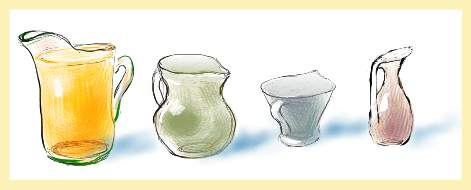#### You may also like### Prompt Cards

These two group activities use mathematical reasoning - one is numerical, one geometric.### Consecutive Numbers

An investigation involving adding and subtracting sets of consecutive numbers. Lots to find out, lots to explore.### Exploring Wild & Wonderful Number Patterns

EWWNP means Exploring Wild and Wonderful Number Patterns Created by Yourself! Investigate what happens if we create number patterns using some simple rules.

# Pouring the Punch Drink

##### Age 7 to 11Challenge Level

a) There are four jugs.

The largest holds exactly $9$ litres of drink, and is filled to the top.

The $7$ litre, $4$ litre and $2$ litre jugs are empty.

Find a way to pour the drink from one jug to another until you are left with exactly $3$ litres in three of the jugs.

b) You have three jugs one of which is full and holds $8$ litres.

The capacity of other jugs is not known. But, it is known that when using them every whole number quantity from $1$ litre to $8$ litres can be accurately measured out.

What could be the capacities of the $2$ other jugs?

How would you measure all the whole number quantities from $1$ to $8$ litres?